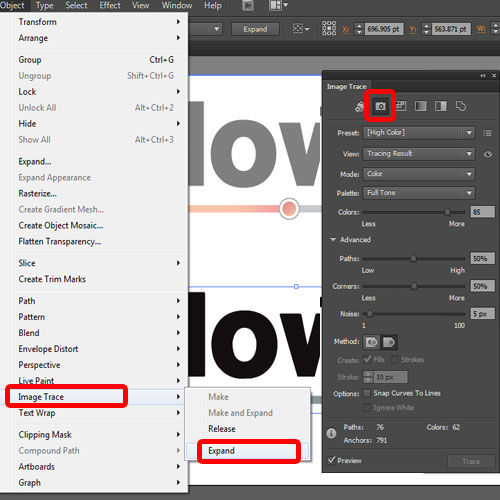What is a Vector Image

What is a vector image? And how is it different from a rasterized image? These are some of the questions we will be answering in this tutorial.

Step 1 – Introducing Vector Images
In the screenshot below, we have two images on the canvas. Notice that we have made a selection of both the objects and that the object on the top is made up of anchor points. That is basically a vector image. Each and every curve, each and every line and each and every direction are represented by an anchor point.
The image below is the same as the image above, but since it’s a rasterized image, it is treated as one single object.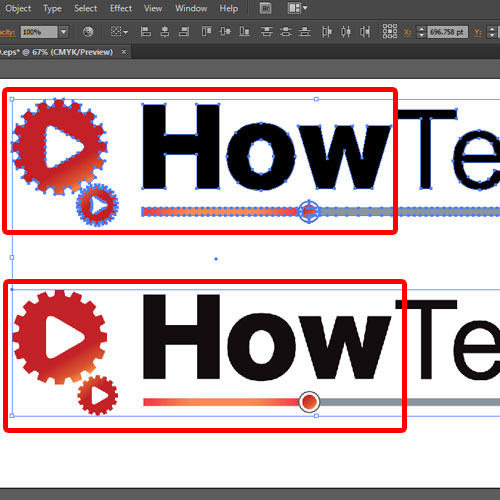Step 2 – Vector vs. Rasterized Image
So what is a vector image, and what makes it different from rasterized images?
If we expand the image below, you will notice that it is made up of pixels. Therefore, when the size of the image is increased, the pixels become clearly visible, and therefore, the image gets distorted.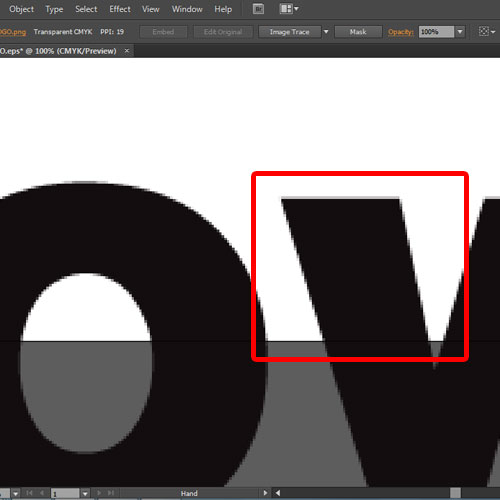Step 3 – Vector Image on Expansion
On the other hand, if we zoom in the vector object, you will notice that the curves are smooth. This is because vector images are drawn using lines and curves based on mathematical equations and control points. These describe the image according to its geometric characteristics.
The vector objects you create using the drawing tools in Adobe Illustrator are examples of vector graphics.
Therefore, the image can be expanded manifolds and the artwork would remain as it is.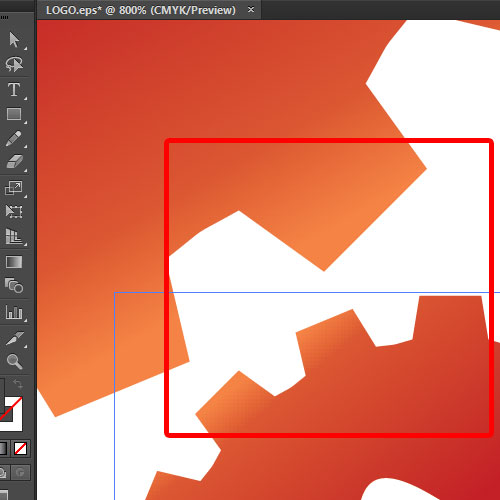Step 4 – Vector Image Editor
Adobe Illustrator is best for working with vector images. You can edit each and every point on the artwork, change the color and move the objects according to your requirements.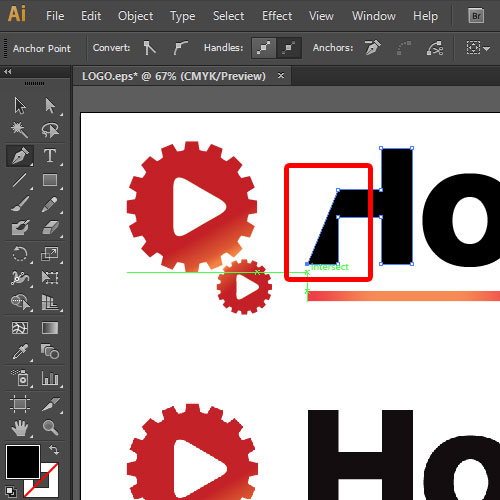Step 5 – Transparency in Vectors
When talking about other features such as transparency, both vector and rasterized images are treated the same way. The only difference is that in a vector image, you can reduce the transparency of each and every component of the artwork individually, while for a rasterized image, you can only change the transparency of the whole image.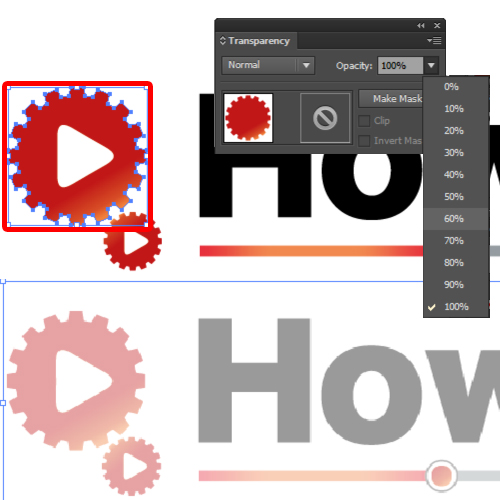Step 6 – Illustrator and Rasterized Images
But when we come back to the rasterized image, you would notice that Adobe Illustrator treats it as one single object. Therefore the artwork itself cannot be edited, you can only move around the object on the canvas.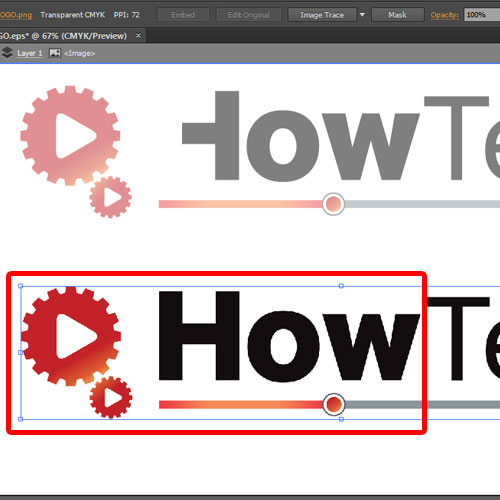Step 7 – Rasterized to Vector Image
Alternatively, you can open up the image trace window which will help you convert a rasterized image into a vector object. You can use the many options in Illustrator, for example the High Color feature to trace an image. After that, if you expand the object from the Image trace menu, you will notice that the artwork will be editable.
Hope you found this basic introduction helpful.